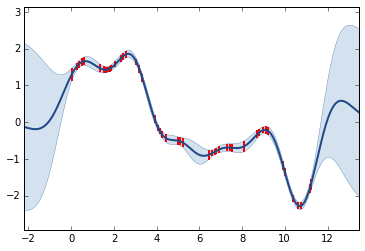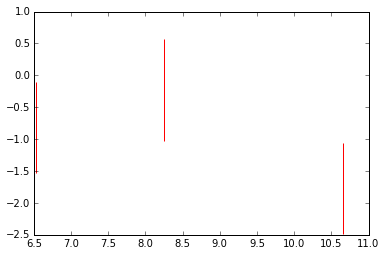# Gaussian Process Summer School, Melbourne, Australia¶

### written by Neil D. Lawrence and James Hensman¶

In this lab we are going to consider low rank approximations to Gaussian process models.

In :
%matplotlib inline
import numpy as np
import matplotlib.pyplot as plt
import GPy
import pods

Populating the interactive namespace from numpy and matplotlib


## Low Rank Approximations¶

In the worst case, inference in a Gaussian process is $\mathcal{O}(n^3)$ computational complexity and $\mathcal{O}(n^2)$ storage. For efficient inference in larger data sets we need to consider approximations. One approach is low rank approximation of the covariance matrix (also known as sparse approximations or perhaps more accurately parsimonious approximations). We'll study these approximations by first creating a simple data set by sampling from a GP.

In :
X = np.sort(np.random.rand(50,1)*12,0)
k = GPy.kern.RBF(1)
K = k.K(X)
K+= np.eye(50)*0.01 # add some independence (noise) to K
y = np.random.multivariate_normal(np.zeros(50), K).reshape(50,1)


Build a straightforward GP model of our simulation. We’ll also plot the posterior of $f$.

In :
m = GPy.models.GPRegression(X,y)
m.optimize()
fig = plt.figure()
m.plot_f(ax=ax)
m._raw_predict?
mu, var = m._raw_predict(X) # this fetches the posterior of f

plt.vlines(X[:,0], mu[:,0]-2.*np.sqrt(var[:,0]), mu[:,0]+2.*np.sqrt(var[:,0]),color='r',lw=2)

Out:
<matplotlib.collections.LineCollection at 0x1115ec990>### Exercise 1¶

One thought that occurs is as follows. Do we need all the data to create this posterior estimate? Are any of the data points redundant? What happens to the model if you remove some data?

Hint:

X2 = np.delete(X,range(8),0)
y2 = np.delete(y,range(8),0)

In :
# Exercise 2 answer here


### Building the Low Rank Approximation¶

Now we’ll consider a GP that uses a low rank approximation to fit the data.

In :
from IPython.display import display
Z = np.random.rand(3,1)*12
m = GPy.models.SparseGPRegression(X,y,Z=Z)
display(m)

creating /var/folders/22/6ls22g994bdfdpwx4f9gcmsw0000gn/T/scipy-neil-tKO4Yo/python27_intermediate/compiler_08f57918657bc3b6af9ef49d104a532a
clang: warning: argument unused during compilation: '-fopenmp'
In file included from /Users/neil/.cache/scipy/python27_compiled/sc_1790bf65208b11355ffcfd4b65a5f1090.cpp:11:
In file included from /Users/neil/Library/Enthought/Canopy_64bit/User/lib/python2.7/site-packages/scipy/weave/blitz/blitz/array.h:26:
In file included from /Users/neil/Library/Enthought/Canopy_64bit/User/lib/python2.7/site-packages/scipy/weave/blitz/blitz/array-impl.h:37:
/Users/neil/Library/Enthought/Canopy_64bit/User/lib/python2.7/site-packages/scipy/weave/blitz/blitz/range.h:120:34: warning: '&&' within '||' [-Wlogical-op-parentheses]
return ((first_ < last_) && (stride_ == 1) || (first_ == last_));
~~~~~~~~~~~~~~~~~^~~~~~~~~~~~~~~~~ ~~
/Users/neil/Library/Enthought/Canopy_64bit/User/lib/python2.7/site-packages/scipy/weave/blitz/blitz/range.h:120:34: note: place parentheses around the '&&' expression to silence this warning
return ((first_ < last_) && (stride_ == 1) || (first_ == last_));
^
(                                 )
In file included from /Users/neil/.cache/scipy/python27_compiled/sc_1790bf65208b11355ffcfd4b65a5f1090.cpp:23:
In file included from /Users/neil/Library/Enthought/Canopy_64bit/User/lib/python2.7/site-packages/numpy/core/include/numpy/arrayobject.h:4:
In file included from /Users/neil/Library/Enthought/Canopy_64bit/User/lib/python2.7/site-packages/numpy/core/include/numpy/ndarrayobject.h:17:
In file included from /Users/neil/Library/Enthought/Canopy_64bit/User/lib/python2.7/site-packages/numpy/core/include/numpy/ndarraytypes.h:1761:
/Users/neil/Library/Enthought/Canopy_64bit/User/lib/python2.7/site-packages/numpy/core/include/numpy/npy_1_7_deprecated_api.h:15:2: warning: "Using deprecated NumPy API, disable it by "          "#defining NPY_NO_DEPRECATED_API NPY_1_7_API_VERSION" [-W#warnings]
#warning "Using deprecated NumPy API, disable it by " \
^
#include <omp.h>
^
2 warnings and 1 error generated.

Weave compilation failed. Falling back to (slower) numpy implementation



Model: sparse gp mpi
Log-likelihood: -89.7838229455
Number of Parameters: 6

sparse_gp_mpi. Value Constraint Prior Tied to
inducing inputs (3, 1)
rbf.variance 1.0 +ve
rbf.lengthscale 1.0 +ve
Gaussian_noise.variance 1.0 +ve

In GPy, the sparse inputs $\mathbf{Z}$ are abbreviated 'iip' , for inducing input. Plot the posterior of $u$ in the same manner as for the full GP:

In :
mu, var = m._raw_predict(Z)
plt.vlines(Z[:,0], mu[:,0]-2.*np.sqrt(var[:,0]), mu[:,0]+2.*np.sqrt(var[:,0]),color='r')

Out:
<matplotlib.collections.LineCollection at 0x1115b99d0>### Exercise 2¶

a) Optimise and plot the model. The inducing inputs are marked – how are they placed? You can move them around with e.g. m['iip_2_0'] = 100 . What happens to the likelihood? What happens to the fit if you remove an input?

In :
# Exercise 3 a answer


b) How does the fit of the sparse compare with the full GP? Play around with the number of inducing inputs, the fit should improve as $M$ increases. How many inducing points are needed? What do you think happens in higher dimensions?